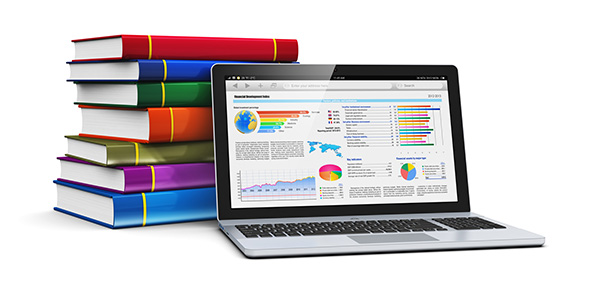# 6:3:1 Sa Of Prisms & Pyramids

4 Questions | Total Attempts: 32SettingsThis quiz will help you prepare for the module 6 test. If necessary: round your answer to the nearest hundredth and/or use the pi key on your calculator.

• 1.
Calculate the surface area of the following figure:If necessary: round your answer to the nearest hundredth and/or use the pi key on your calculator.
• A.

36 square cm

• B.

68 square cm

• C.

36 cubic cm

• D.

68 cubic cm

• 2.
Calculate the surface area of the following figure:If necessary: round your answer to the nearest hundredth and/or use the pi key on your calculator.
• A.

184.5 square m

• B.

160.5 square m

• C.

190.5 square m

• D.

211.3 square m

• 3.
Calculate the surface area of the following figure:If necessary: round your answer to the nearest hundredth and/or use the pi key on your calculator.
• A.

35.2 square cm

• B.

55.7 square cm

• C.

70 square cm

• D.

87.2 square cm

• 4.
Calculate the surface area of the following figure:If necessary: round your answer to the nearest hundredth and/or use the pi key on your calculator.
• A.

324 square units

• B.

108 square units

• C.

5832 square units

• D.

1944 square units